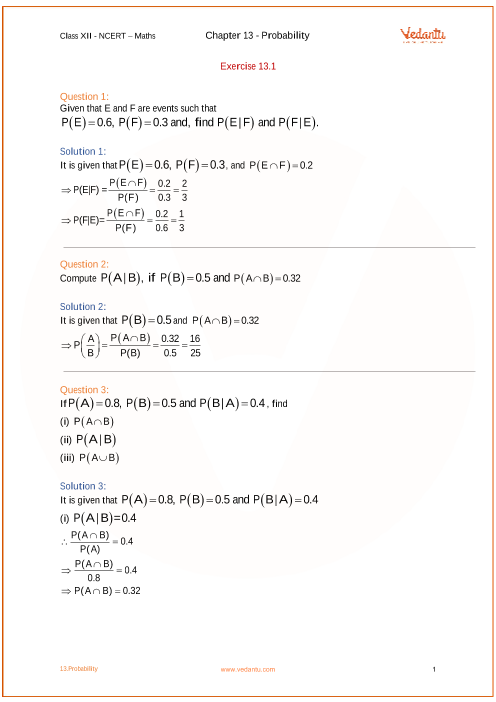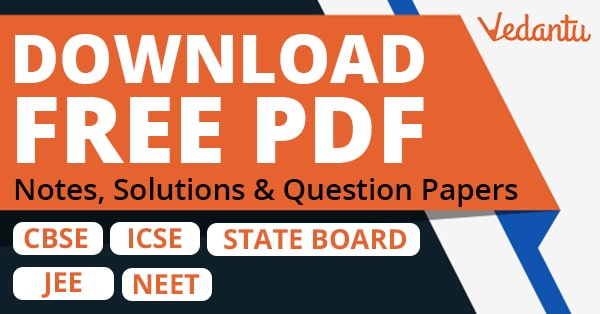NCERT Solutions for Class 12 Maths Chapter 13 Probability (Ex 13.3) Exercise 13.3

NCERT Solutions for Class 12 Maths Chapter 13 Probability (Ex 13.3) Exercise 13.3

Free PDF download of NCERT Solutions for Class 12 Maths Chapter 13 Exercise 13.3 (Ex 13.3) and all chapter exercises at one place prepared by expert teacher as per NCERT (CBSE) books guidelines. Class 12 Maths Chapter 13 Probability Exercise 13.3 Questions with Solutions to help you to revise complete Syllabus and Score More marks. Register and get all exercise solutions in your emails.